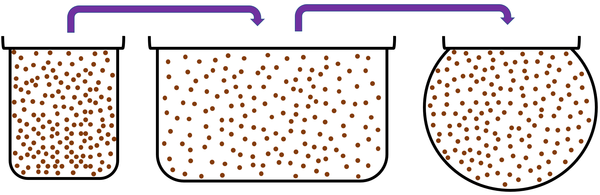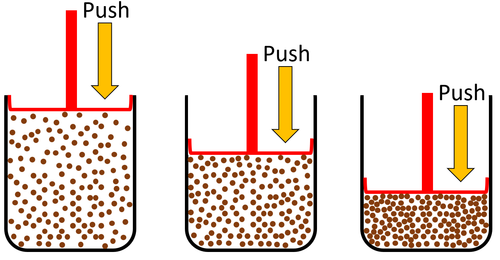# Gas

## Key Stage 2

### Meaning

Gas is a state of matter that can change size and shape to fit any container.

Most gases are invisible but we can feel them.
When the air moves we call it the wind.Cannot hold their shape. Fit the shape of their container. Can be poured and will flow.Gases can be squashed into a smaller size.

Examples of gas materials:

• Air (A mixture of gases, mostly nitrogen and oxygen)
• Steam

## Key Stage 3

### Meaning

Gas is a State of Matter in which the particles are separated by large distances and can move freely.Gases cannot hold their shape because the particles are free to move. Gases fit the shape of their containers because the particles are free to move. A gas can be poured and will flow because the particles are free to move.Gas can be squashed into a smaller size because the particles are spread apart.

## Key Stage 4 Foundation

### Meaning

Gas is a State of Matter in which the particles are separated by large distances and can move freely.

When a substance is in its gaseous state it is always less dense than in its liquid or solid state due to the particles in a gas being spread far apart from each other.
A substance which is gaseous at room temperature has a smaller force of attraction between particles than a substance which is liquid or solid at room temperature.
 Particle Diagram Particle Arrangement PropertyParticles are free to move in all directions. Gases fit the size of their container. Gases fit the shape of their container. Convection happens most easily in gases. Particles are spread apart. Gases can be compressed into a smaller volume. Sound passes through gases slower than liquids and solids. Thermal Conduction is very poor in a gases.

## Key Stage 4 Higher

### Molar Gas Volume

At Standard Temperature and Pressure (20°C and 101,000Pa) the volume of one mole of gas is 24 dm3.
The volume of gas required or produced in a chemical reaction can be calculated from the mass of the gas and its Relative Formula Mass.

#### Example Calculations

 Calculate the volume of 4g of Hydrogen gas. Calculate the volume of 8g of Oxygen gas. Calculate the volume of 20g of Methane gas. 1.Find the Relative Formula Mass of the gas. Chemical Formula of Hydrogen = H2 Relative Formula Mass of H2 = 2g 1.Find the Relative Formula Mass of the gas. Chemical Formula of Oxygen = O2 Relative Atomic Mass of Oxygen = 16g Relative Formula Mass of O2 = 32g 1.Find the Relative Formula Mass of the gas. Chemical Formula of Methane = CH4 Relative Atomic Mass of Carbon = 12g Relative Formula Mass of CH4 = 16g 2. Calculate the number of moles of gas. 1 mole = 2g No. Moles = $$\frac{Mass}{M_r}$$ No. Moles = $$\frac{4}{2}$$ No. Moles = 2mol 2. Calculate the number of moles of gas. 1 mole = 32g No. Moles = $$\frac{Mass}{M_r}$$ No. Moles = $$\frac{8}{32}$$ No. Moles = 0.25 mol 2. Calculate the number of moles of gas. 1 mole = 16g No. Moles = $$\frac{Mass}{M_r}$$ No. Moles = $$\frac{20}{16}$$ No. Moles = 1.25 mol 3. Find the volume in dm3. Volume = 24 x (number of moles) Volume = 24 x (2) Volume = 48dm3 3. Find the volume in dm3. Volume = 24 x (number of moles) Volume = 24 x (0.25) Volume = 6dm3 3. Find the volume in dm3. Volume = 24 x (number of moles) Volume = 24 x (1.25) Volume = 30dm3
 Calculate the mass of 12dm3 of Hydrogen gas. Calculate the mass of 36dm3 of Nitrogen gas. Calculate the mass of 18dm3 of Ethane gas. 1.Find the Relative Formula Mass of the gas. Chemical Formula of Hydrogen = H2 Relative Formula Mass of H2 = 2g 1.Find the Relative Formula Mass of the gas. Chemical Formula of Nitrogen = N2 Relative Atomic Mass of Nitrogen = 14g Relative Formula Mass of N2 = 28g 1.Find the Relative Formula Mass of the gas. Chemical Formula of Ethane = C2H6 Relative Atomic Mass of Carbon = 12g Relative Formula Mass of C2H6 = 30g 2. Calculate the number of moles of gas. Volume = 24 x (number of moles) 12 = 24 x (number of moles) Number of moles = 12/24 Number of moles = 0.5 2. Calculate the number of moles of gas. Volume = 24 x (number of moles) 36 = 24 x (number of moles) Number of moles = 36/24 Number of moles = 1.5 2. Calculate the number of moles of gas. Volume = 24 x (number of moles) 12 = 24 x (number of moles) Number of moles = 18/24 Number of moles = 0.75 3. Find the mass of gas from the number of moles. No. Moles = $$\frac{Mass}{M_r}$$ 0.5 = $$\frac{Mass}{2}$$ Mass = $$0.5\times2$$ Mass = 1g Volume = 48dm3 3. Find the mass of gas from the number of moles. No. Moles = $$\frac{Mass}{M_r}$$ 1.5 = $$\frac{Mass}{28}$$ Mass = $$1.5\times28$$ Mass = 42g 3. Find the mass of gas from the number of moles. No. Moles = $$\frac{Mass}{M_r}$$ 0.75 = $$\frac{Mass}{30}$$ Mass = $$0.75\times30$$ Mass = 22.5g